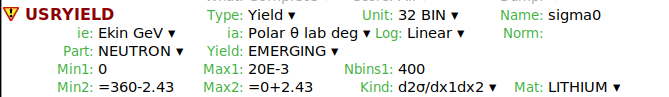# Invalid angular limits for Usryld

Dear expert,

In this input, I tried to get information from USRYIELD card in 0 degree lab angle with an angular deviation of ± 2.43. But I got an error

*** Invalid angular limits for Usryld:
In this case, do I have to use 360 - 2.43 and 0+ 2.43 ?Regards,

Riya

If you want to look at 0 deg with an acceptance of 2.43 deg, you have to put Min2=0 and Max2=2.43.
Here you wrote instead from 357.57 to 2.43, which makes no sense since the polar angle cannot exceed 180 deg. Note that, if you consider for instance the xz plane, what you have in mind as -2.43 deg (i.e. a direction in the negative-x halfplane) actually corresponds to a polar angle (theta) of +2.43 deg and an azimuthal angle (phi) of 180 deg, and is automatically taken into account in the polar angle interval I indicated above since USRYIELD scores over the whole azimuthal angle interval of 360 deg.

Thank you @ceruttif for clarifying it. Just to understand this properly, for other angles I was doing min = 𝜃_1 - 𝑑𝜃_1 and max = 𝜃_1 + 𝑑𝜃_1. But this is then wrong, I guess. I have to use min = 𝜃_1 and max = 𝜃_1 + 𝑑𝜃_1. [Since for 0 degree angle, we have to use 0 to 0 + 2.43]. Can you please clarify this?

Regards,
Riya

No, it was correct, since theta=0 is a special case. However, your drawing for theta1 is wrong, since the azimuthal angle (running from 0 to 360 deg) shall remain in the plane orthogonal to the z-axis (as for theta0), giving in this case a hollow surface. Refer to the spherical coordinate definition (in Wikipedia or any relevant textbook).

Dear @ceruttif ,

Thank you for the clarification. Yes, this image is not correctly drawn, I meant a configuration described here.

Regards,
Riya

Also that figure does not represent the USRYIELD scoring reality, since USRYIELD scores over the whole azimuthal angle interval of 360 deg, contrary to what is shown there, which is limited to a small azimuthal angle interval, as for a typical detector. Nonetheless, once divided by the (respective) solid angle, (experimental and simulated) results can be compared. In fact, USRYIELD exploits the azimuthal symmetry and scores not only within the physical surface of the detector, but over the whole 360 deg azimuthal range, gaining this way much more statistics. As just mentioned, the fact that results are given as /dOmega provides a clean quantity.

Thank you @ceruttif for the detailed explanation.

Regards,

Riya